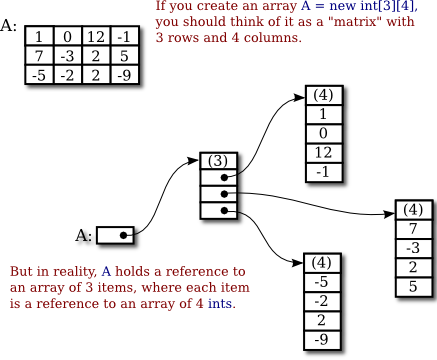# Handling with Multi-Dimentional Variable Arrays in Java

I want to be straightforward on this topic on how to Handle Multi-Dimentional Variable Arrays in Java.

This variable array, composed of 2 rows and 5 columns as you can see below

```// int[][]  A  =  new int;
```

can be initial initialize with the following array values

```int[][]  A  =  {  {  1,  0, 12, -1, 9 }, // First Row
{  7, -3,  2,  5, 10 } // Second Row
};
```

Here’s the better figure for your deeper comprehension.Source: http://math.hws.edu/javanotes/c7/s5.html

But this time, I am dealing with 2 x 5 matrix.

## Printing Individual Variable Value

To print a particular a particular element from Row 1, let say we want to print “12”,
Just add this line after variable declaration

```System.out.println(A) //  means, First Row and  means 3rd element in the First Row
// This will print "12"
```

## Printing Values in Each Rows and Columns

```public class TwoDimentionalArray {

public static void main( String args[] ) {
try {
//	int[][]  A  =  new int;

int[][]  A  =  {  {  1,  0, 12, -1, 9 },
{  7, -3,  2,  5, 10 }
};

for (int i = 0; i < A.length; i++) {

TextIO.put(A[i] + "\t");  // Printing all values in the First Row
TextIO.putln(A[i]);       // Printing all values in the Second Row

}

} catch ( ArrayIndexOutOfBoundsException e ) {TextIO.putln("Exceeded!"); }

}
}
```

## To Handle all Variable Arrays in one line

```public class ArrayMultidimensional {
public static void main( String args[] ) {
try {
//	int[][]  A  =  new int;

int[][]  A  =  {  {  1,  0, 12, -1, 9 },
{  7, -3,  2,  5, 10 }
};

for (int i = 0; i < A.length; i++) {
for (int j = 0; j < 5; j++) {
TextIO.putln( A[i][j]);
}

}

} catch ( ArrayIndexOutOfBoundsException e ) {System.out.println("Exceed"); }

}
}

```

Storing values in Variable arrays will save you time to handle many variables. In Java, there’s no other method on dealing with large variables. I used to program using TextIO, which you can also replace it with System.out.print as a native class in Java package.Elvin is one of the contributors of mylabplus.com. He has been creating Wordpress Plugins, Magento Extensions, & Mobile Applications. If you find this article useful, please Follow him on .